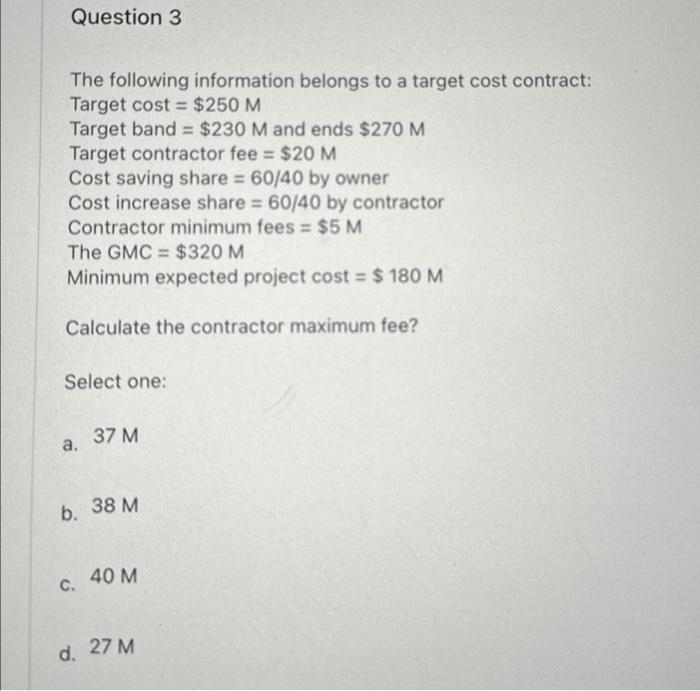Home / Expert Answers / Civil Engineering / question-3-the-following-information-belongs-to-a-target-cost-contract-target-cost-250-m-target-pa234

# (Solved): Question 3 The following information belongs to a target cost contract: Target cost = $250 M Target ... Question 3 The following information belongs to a target cost contract: Target cost =$250 M Target band = $230 M and ends$270 M Target contractor fee = $20 M Cost saving share = 60/40 by owner Cost increase share = 60/40 by contractor Contractor minimum fees =$5 M The GMC = $320 M Minimum expected project cost =$ 180 M Calculate the contractor maximum fee? Select one: a. b. 38 M C. 37 M d. 40 M 27 MThe following information belongs to a target cost contract: Target cost Target band and ends Target contractor fee Cost saving share by owner Cost increase share by contractor Contractor minimum fees The Minimum expected project cost Calculate the contractor maximum fee? Select one: a. b. c. d.

We have an Answer from Expert